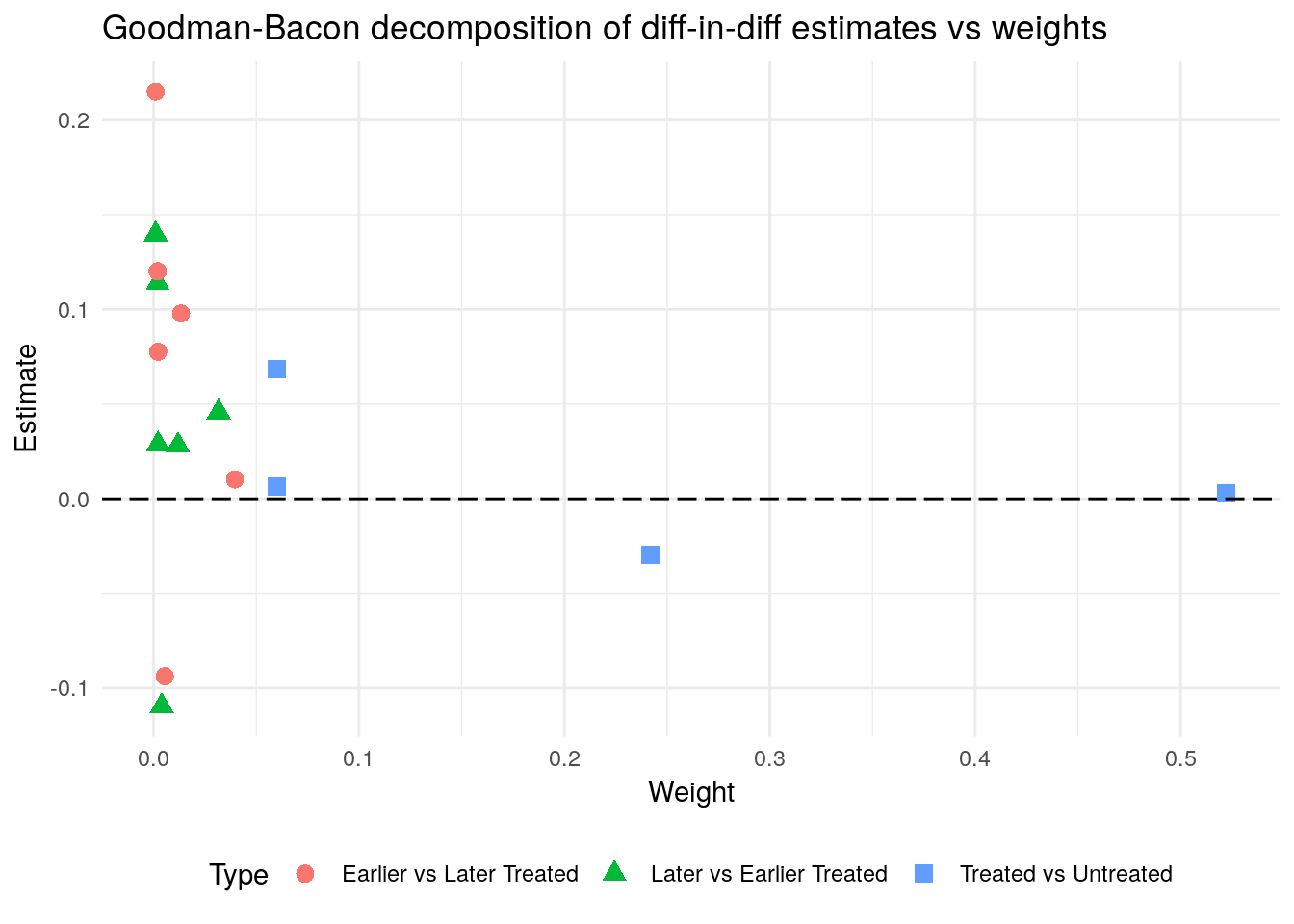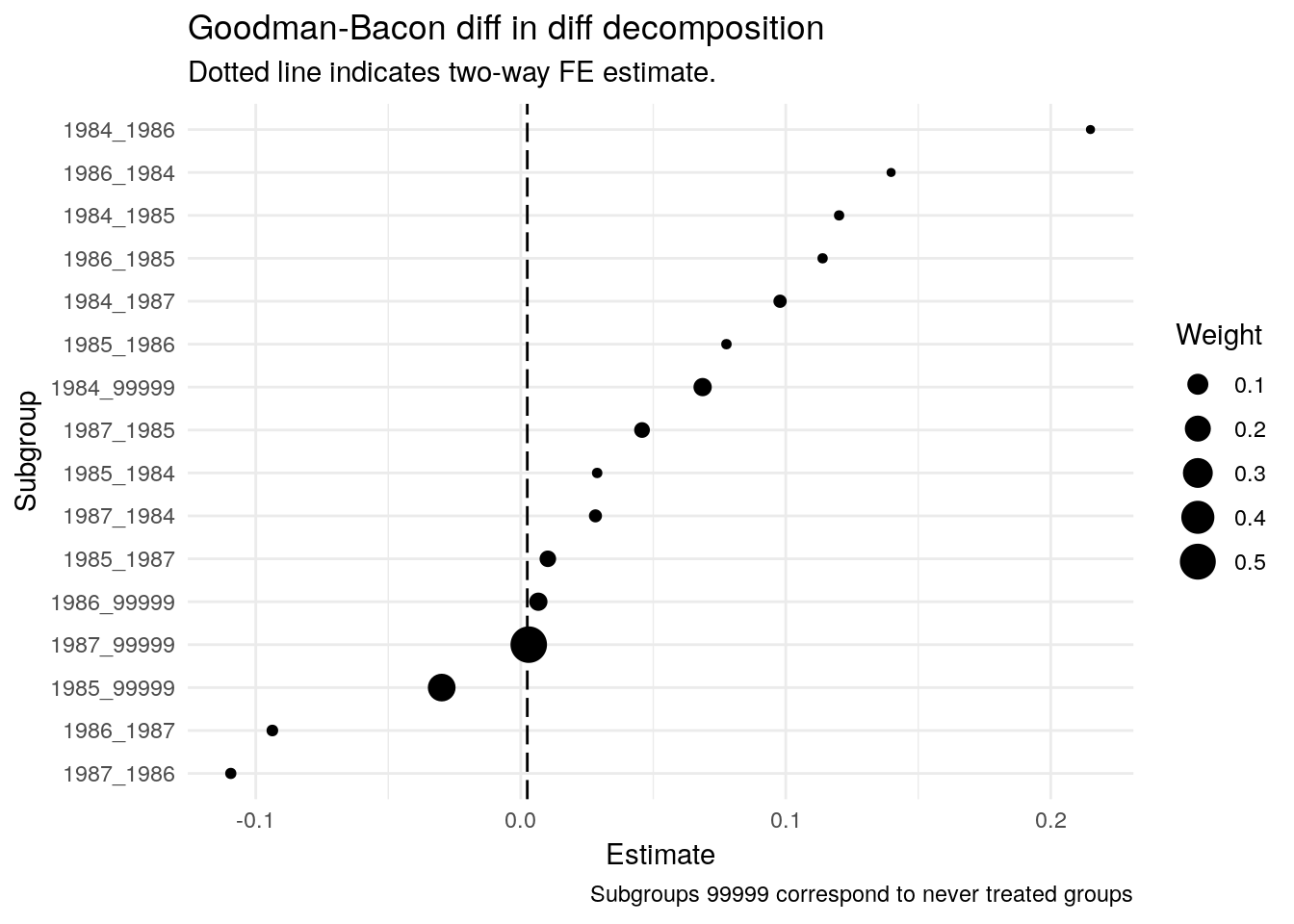Evan and I have released an R package bacon to perform the Goodman-Bacon decomposition. The paper decomposes diff-in-diff estimates with time-varying treatment into a set of “two-by-twos” comparing treated and untreated groups at each timing stage. Evan did 99% of the work and it was great fun collaborating on an open source project with him. Below is a very quick example of how the package works.

Installing the package from GitHub (we’re not on CRAN just yet) and loading libraries:

devtools::install_github("evanjflack/bacon")
library(bacon)
library(ggplot2)
library(dplyr)

Perform the decomposition and plot estimates vs weights, using the default math_reform dataset included in the package:

df_bacon <- bacon(incearn_ln ~ reform_math,
data = bacon::math_reform,
id_var = "state",
time_var = "class")

ggplot(df_bacon) +
aes(x = weight,
y = estimate,
shape = factor(type),
colour = factor(type)) +
geom_point(size = 3) +
geom_hline(yintercept = 0,
linetype = "longdash") +
labs(x = "Weight",
y = "Estimate",
shape = "Type",
colour = "Type",
title = "Goodman-Bacon decomposition of diff-in-diff estimates vs weights") +
theme_minimal() +
theme(legend.position = "bottom")Plotting estimates from largest to smallest alongside the traditional two-way FE estimate:

df_bacon %>%
mutate(subgroup = paste0(treated, "_", untreated),
subgroup = factor(subgroup),
subgroup = forcats::fct_reorder(subgroup, estimate)) %>%
ggplot(aes(x = estimate,
y = subgroup,
size = weight)) +
geom_point() +
geom_vline(xintercept = weighted.mean(df_bacon$estimate, df_bacon$weight),
linetype = "longdash") +
theme_minimal() +
labs(size = "Weight",
y = "Subgroup",
x = "Estimate",
title = "Goodman-Bacon diff in diff decomposition",
subtitle = "Dotted line indicates two-way FE estimate.",
caption = "Subgroups 99999 correspond to never treated groups")We’re always open to feedback and suggestions - just open a GitHub issue.